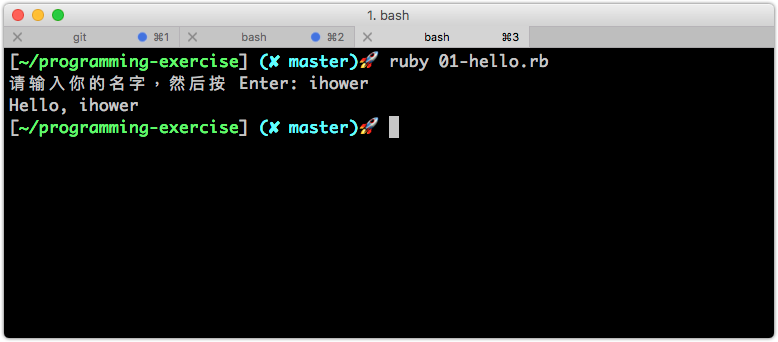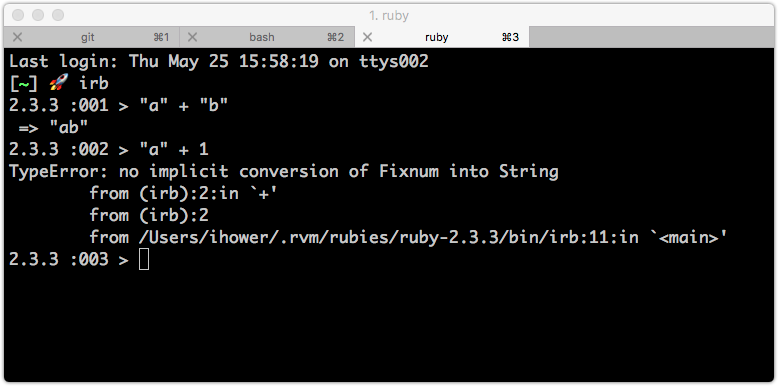# 1. 前言準備

1. 輸入、處理、輸出
2. 基本算術
3. 控制流程
4. 函式方法
5. 循環
6. 陣列 Array
7. 雜湊 Hash
8. 物件 Object
9. 檔案 File

`git clone git@github.com:your_name/programming-exercise.git` `cd programming-exercise.git`

`ruby 01-hello.rb`

# 2. 輸入、處理、輸出

## 題目 1## 提示說明

### 標準螢幕輸出(STDOUT)

• `puts` 會輸出變數在螢幕上，並且有換行
• `print` 也會輸出變數在螢幕，不會換行

### 標準螢幕輸入(STDIN)

`gets` 可以從鍵盤拿到輸入的值。

### 字串 String

• `"用雙引號包起來"`
• `'或是用單引號'`
• `"如果裡面有'單引號'，那就要用雙引號包起來"`
• `'如果裡面有"雙引號"，那就要用單引號包起來'`
• `"如果你堅持雙引號裡面要有雙引號，那你得寫成 \" 做逸出"`

### 字串串接

`+` 的，例如 `"a" + "b"`

### 字串內嵌

`#{}` 的語法可以將變數內嵌在字串中

### irb 互動式 Ruby 環境# 3. 基本算術

## 提示說明

### 什麽是數據類型？

• 字串 String
• 整數 Integer (不可以有小數)
• 浮點數 Float (可以存放小數)
• 陣列 Array
• 雜湊 Hash

### 整數和浮點數

• 沒有小數點的就是整數，例如 `123`
• 有小數點的就是浮點數，例如 `123.0``12.3`

### 有哪些基本算術?

• `+`
• `-`
• `*`
• `/`
• `%` 餘數，例如 `20 % 3``2`

### 型別轉換 (Type Conversion)

• 透過 `to_i` 可以將字串轉成整數，例如 `"123".to_i` 變成 `123`
• 透過 `to_f` 可以將整數轉乘浮點數，例如 `123.to_f` 變成 `123.0`
• 透過 `to_s` 可以轉成字串，例如 `123.to_s` 變成 `"123"`

### 浮點數常用方法

``````1.4.round      #=> 1
1.5.round      #=> 2
1.6.round      #=> 2
(-1.5).round   #=> -2
``````

``````1.2.ceil      #=> 2
2.0.ceil      #=> 2
(-1.2).ceil   #=> -1
(-2.0).ceil   #=> -2
``````

``````1.2.floor      #=> 1
2.0.floor      #=> 2
(-1.2).floor   #=> -2
(-2.0).floor   #=> -2
``````

# 4. 控制流程

## 題目 5

BMI 公式為 bmi = ( 體重 / (身高x身高) )，單位是公斤和公尺

• 如果 BMI < 18.5，顯示過輕
• 如果 BMI >= 24，顯示過重
• 如果 BMI 介於 18.5 ~ 24，顯示正常

## 題目 7

• 當 x < 0 輸出 “A”
• 當 x > 0
• 當 y > 0
• 當 z > 0 輸出 “B”
• 當 z < 0 輸出 “C”
• 當 y < 0
• 當 z > 0 輸出 “D”
• 當 z < 0 輸出 “E”

## 提示說明

• `==`
• `!=`
• `<`
• `>`
• `<=`
• `>=`

### 控制流程(Control Flow)

``````if 9 > 3
# 這段會執行
else
# 這段不會執行
end

``````

``````if a > b
# ...
elsif a == b
# ...
else
# ...
end

``````

### 邏輯運算子

`&&`

• `a``b` 都是 `true` 時，`a && b` 回傳 `true`
• `a``b` 任一個是 `false` 時，`a && b` 回傳 `false`

`||`

• `a``b` 任一個是 `true` 時，`a || b` 回傳 `true`
• `a``b` 都是 `false` 時，`a || b` 回傳 `false`

`!` 相反

``````if ( 9 > 3 ) && ( 6 == 6)
# ...
elsif ( 2 > 1 ) || ( 5 < 1 )
# ...
end

``````

## Boolean 轉型

``````if "foobar"
# 會執行
end

``````
``````if 123
# 會執行
end
``````
``````if 0
# 會執行
end
``````
``````if ""
# 會執行
end
``````
``````if []
# 會執行
end
``````
``````if nil
# 不會執行
end
``````

# 5. 方法 Method

## 提示說明

### 如何定義方法和調用

`def`

``````def hello
puts "Hello"
end

hello()  # 這樣會調用 hello 方法

hello    # 在 Ruby 語言中 () 可以省略
``````

### 如何帶入參數

``````def hello(name)
puts "Hello, #{name}"
end

hello("ihower")   # 這樣會調用 hello 方法

hello "ihower"    # 在 Ruby 語言中 () 可以省略

``````

### 回傳值

``````def get_hello(name)
return "Hello, #{name}"
end

str = get_hello("ihower")
puts str

``````

``````def get_hello(name)
"Hello, #{name}"
end

``````

# 6. 循環 Loop

## 題目 13

``````0 x 0 = 0
0 x 1 = 0
......
12 x 11 = 132
12 x 12 = 144
``````

## 提示說明

### 什麽是循環？

``````x = 10
while x <= 10
puts x
x = x + 1
end

``````

### 提前中斷

`while` 循環中，用 `break` 關鍵字，會提前中斷循環

# 7. 陣列 Array

## 題目 21

Ruby 的 Array 陣列是內建了 `.sort` 方法可排序，我們這裡練習自行實作一個排序算法。

## 提示說明

### 什麽是陣列？

• 一個有順序的容器，用數字當作索引。
• 索引從 0 開始
• 原則上裡面的元素，數據類型最好都是一樣的，例如都存整數，或是都存字串。

``````array = [5,1,2,3,5]
``````

``````array = [
[1,2,3],
[4,5,6],
[7,8,9]
]

array # 這是 1
array # 這是 2
array # 這是 9
``````

### 如何走訪陣列？

`for` 方法：

``````for i in array
puts i
end
``````

``````array.each do |i|
puts i
end

``````

``````array.each_with_index do |i, j|
puts i
puts j   # j 從 0 開始數
end

``````

### 如何操作陣列？

`array.size` 會回傳陣列長度

`array` 會讀取第二個元素

`array = 999` 會更改第二的元素的值

`array` 讀取一個不存在的值，會回傳 `nil`

`array``array.first` 是陣列的第一個元素

`array[-1]``array.last` 是陣列的最後一個元素

`array.push(999)``array << 999` 會新增一個元素 999 到陣列的最後面

`array.unshift(999)` 會新增一個元素 999 到陣列的最前面

`array.delete_at(i)` 會刪除索引值在 i 的元素

### 字串與陣列

``````str = "ABCDEFG"

str  # 得到 "A"

str = "x"
str   # 變成 "AxCDEFG"

str.split("") # 用 "" 拆開字串，得到陣列 ["A", "B", "C", "D", "E", "F", "G"]

["x", "y", "z"].join(" ") # 用 " " 串接成字串，得到 "x y z"

``````

# 8. 雜湊 Hash

## 提示說明

### 什麽是雜湊 Hash？

`h = { "a" => 123, "b" => 456 }`

`h["a"]` 就是 123

`h["b"]` 就是 456

`h["qweqkleklwqen"]` 如果讀取一個不存在的key，結果會是 nil

`h["new_key"] = 123` 如果本來沒有這個 key，就會直接新增一組 key-value

### 如何走訪雜湊 Hash？

``````h.each do |key, value|
puts key
puts value
end

``````

### 常用方法

`h.keys` 會回傳一個陣列包括所有 keys

`h.values` 會回傳一個陣列包括所有 values

`h.merge(h2)` 會合並雜湊 h2 到雜湊 h

# 9. 物件 Object

## 提示說明

### 什麽是物件?

`"abc".size` 會回傳 3

`"a,b,c,d".split(",")` 會回傳陣列 `["a", "b", "c", "d"]`

### 如何自訂義物件?

``````class Car

attr_accessor :color  # 這會定義屬性 @color

def run
puts "This #{@color} car is running"
end
end

``````

``````car1 = Car.new
car1.color = "red"
car1.run

car2 = Car.new
car2.color = "blue"
car2.run

``````

### 內建的類別

`"foobar".class` 會回傳 `String` 是個類別

`String.new("foobar")` 等同於 `"foobar"`

`[1,2,3].class` 會回傳 `Array` 是個類別

`Array.new([1,2,3])` 等同於 `[1,2,3]`

### 什麽是常數 (Constant)？

`STATUS = ["pending", "confirmed"]`

`Pi = 3.1415926`

# 10. 檔案處理

## 提示說明

### 如何開檔讀取和寫入

``````file = File.open("foo.txt")

``````

``````File.open("bar.txt", "w+") do |f|
f << "aaa"
f << "\n"
f << "bbb"
end
``````

``````aaa
bbb

``````